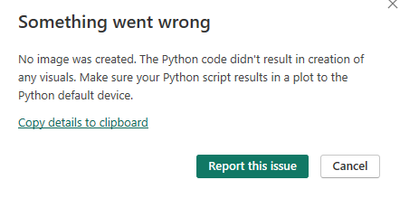cancel
Showing results for
Did you mean:Regular Visitor

## Python script for Power BI Desktop visualization does not work

Hello, everyone!

Now I am studying Python and as I previously worked with Power BI, I tried to test how it would be to run Python vizualization script in Power BI Desktop.

The script I tried to run is written using Python plotly library and works perfect on Jupyter and there is nothing special about it:

import pandas as pd

import matplotlib.pyplot as plt

import plotly.express as px

import plotly.graph_objects as go

df['open_time_timeformat'] = pd.to_datetime(df['open_time'], unit='ms').dt.strftime('%Y-%m-%d %H:%M:%S')

df['close_time_timeformat'] = pd.to_datetime(df['close_time'], unit='ms').dt.strftime('%Y-%m-%d %H:%M:%S')

df['day'] = pd.to_datetime(df['close_time'], unit='ms').dt.strftime('%d')

fig = go.Figure(data=[go.Candlestick(x=df['close_time_timeformat'],

open=df['open'],

high=df['high'],

low=df['low'],

close=df['close'])])

fig.update_layout(hovermode='x unified', autosize = True, title={

'text': "High, low, open and close prices of BTCUSDT",

'y':0.85,

'x':0.5,

'xanchor': 'center',

'yanchor': 'top'})

fig.update_layout(hoverlabel=dict(

bgcolor="white",

font_size=22,

font_family="Rockwell"))

fig.update_yaxes(type="log")

fig.show()

The problem is that after running it in Power BI Desktop Python script editor, it:

1)Does not show visual on PBI –shows error “can’t display visual”2)Internet browser starts trying to open URL page, showing “this site can’t be reached” and few times it even succeeds to open visualization (and shows as perfect as on Jupyter)!!!, which completely is not a purpose of this and script does not provide to do it!?

Could you please advise what would is a problem and is it necessary to do extra manipulations in PBI Desktop for Python script to be working properly?

p.s. I have all necessary Python modules installed and paths are correct in PBI Python scripting options – otherwise it would not be possible to load script into PBI Desktop.

Regards,

0 REPLIES 0